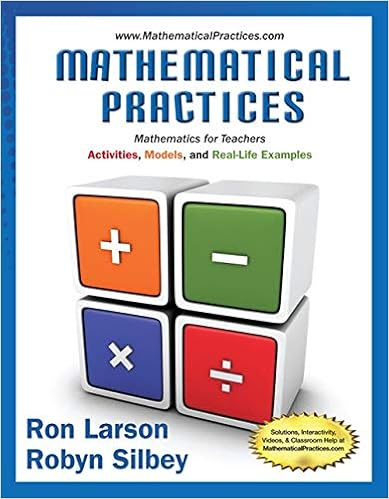• 61
• 99% (67) 66 out of 67 people found this document helpful

This preview shows page 21 - 23 out of 61 pages.

##### We have textbook solutions for you!
The document you are viewing contains questions related to this textbook.The document you are viewing contains questions related to this textbook.
Chapter 4 / Exercise 49
Mathematical Practices, Mathematics for Teachers: Activities, Models, and Real-Life Examples
LarsonExpert Verified
Answer: FALSE Explanation: The variable overhead efficiency variance is computed the same way as the efficiency variance for direct-cost items. Diff: 1 Type: TF
Skill: Remember Objective: LO 8-2 7) The variable overhead flexible-budget variance measures the difference between standard variable overhead costs and flexible-budget variable overhead costs. Diff: 1 Type: TF
Skill: Remember Objective: LO 8-2 8) The variable overhead efficiency variance measures the efficiency with which the cost- allocation base is used. Diff: 2 Type: TF
Skill: Understand Objective: LO 8-2 9) The variable overhead efficiency variance can be interpreted the same way as the efficiency variance for direct-cost items. Diff: 2 Type: TF
Skill: Understand Objective: LO 8-2
##### We have textbook solutions for you!
The document you are viewing contains questions related to this textbook.The document you are viewing contains questions related to this textbook.
Chapter 4 / Exercise 49
Mathematical Practices, Mathematics for Teachers: Activities, Models, and Real-Life Examples
LarsonExpert Verified
10) There is causal relationship between the variable overhead allocation base and the variable overhead cost pool. Diff: 2 Type: TF
Skill: Understand Objective: LO 8-2 11) Effective planning of variable overhead costs means that a company performs those variable overhead costs that primarily add value B Diff: 2 Type: MC
Skill: Understand Objective: LO 8-2 12) Two of the primary ways to manage variable overhead costs include A Diff: 2 Type: MC
Skill: Understand Objective: LO 8-2
•••